Most Affordable JEE | NEET | 8,9,10 Preparation by Kota's Top IITian Doctor Faculties

# NCERT Solutions for Class 11 Chemistry chapter 3 Classification of Elements and periodicity in Properties PDF - eSaral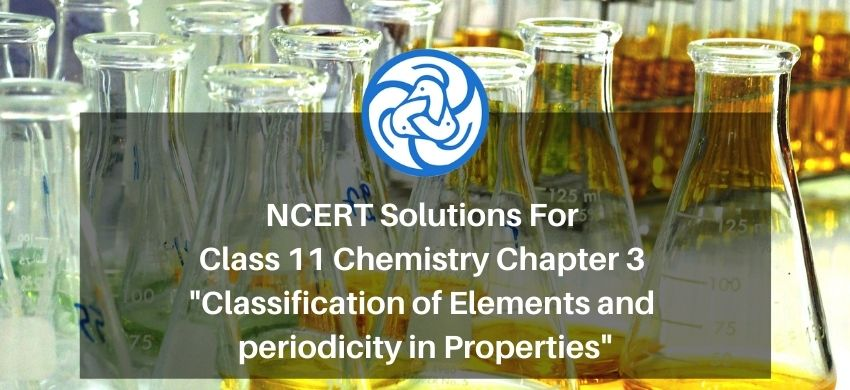`

# NCERT Solutions for Class 11 Chemistry chapter 3 Classification of Elements and periodicity in Properties PDF

Hey, are you a class 11 student and looking for ways to download NCERT Solutions for Class 11 Chemistry chapter 3 Classification of Elements and periodicity in Properties pdf? If yes. Then read this post till the end.

In this article, we have listed NCERT Solutions for Class 11 Chemistry chapter 3 Classification of Elements and periodicity in Properties in PDF that are prepared by Kota’s top IITian’s Faculties by keeping Simplicity in mind.

If you want to learn and understand class 11 Chemistry chapter 3 "Classification of Elements and periodicity in Properties" in an easy way then you can use these solutions PDF.

NCERT Solutions helps students to Practice important concepts of subjects easily. Class 11 Chemistry solutions provide detailed explanations of all the NCERT questions that students can use to clear their doubts instantly.

If you want to score high in your class 11 Chemistry Exam then it is very important for you to have a good knowledge of all the important topics, so to learn and practice those topics you can use eSaral NCERT Solutions.

In this article, we have listed NCERT Solutions for Class 11 Chemistry chapter 3 Classification of Elements and periodicity in Properties pdf that you can download to start your preparations anytime.

So, without wasting more time Let’s start.

## Download NCERT Solutions for Class 11 Chemistry chapter 3 Classification of Elements and periodicity in Properties PDF

Question 1. What is the basic theme of organisation in the periodic table?

Solution: The basic theme of the organisation of elements in the periodic table is that the elements are classified in periods and groups according to their physical and chemical properties. This arrangement makes the study of elements and their compounds simple and systematic. In the periodic table, an element with similar properties is placed in the same group.

Question 2. Which important property did Mendeleev use to classify the elements in his periodic table and did he stick to that?

Solution. Mendeleev arranged the elements in his periodic table according to their atomic weight or mass. He arranged the elements in periods and groups in order of their increasing atomic weight. He also kept the elements with similar properties in the same group. But, he found out that if the elements were arranged strictly in order of their increasing atomic weights, then some elements did not fit within this scheme of classification. Therefore, he ignored the order of atomic weights in some cases. For example, the atomic weight of iodine is lower than that of tellurium. Still, Mendeleev placed tellurium (in Group VI) before iodine (in Group VII) because iodine's properties are so similar to fluorine, chlorine, and bromine.

Question 3. What is the basic difference in approach between the Mendeleev's Periodic Law and the Modern Periodic Law?

Solution. Mendeleev's periodic law states that the physical and chemical properties of elements are periodic functions of their atomic weights. Whereas, the modern periodic law states that the physical and chemical properties of elements are periodic functions of their atomic numbers.

Question 4. On the basis of quantum numbers, justify that the sixth period of the periodic table should have 32 elements.

Solution. In the periodic table of the elements, a period indicates the value of the principal quantum number (n) for the outermost shells. Each period begins with a principal quantum number (n).

The value of $\mathrm{n}$ for the sixth period is 6 . And for $\mathrm{n}=6$, Azimuthal quantum number (1) can have values of $0,1,2,3,4$.

According to Aufbau's principle, electrons are added to different orbitals in order of their increasing energies $[(\mathrm{n}+1)$ rule].

$(\mathrm{n}+\mathrm{l})$ value for $6 \mathrm{~d}$ subshell $=6+2=8$

$(\mathrm{n}+\mathrm{l})$ value for $7 \mathrm{~s}$ subshell $=7+0=7$

The energy of the 6 d subshell is even higher than that of the $7 \mathrm{~s}$ subshell according to $(\mathrm{n}+\mathrm{l})$ rule

In the 6 th period,

$(n+l)$ value for 6 s subshell $=6+0=6$

$(n+l)$ value for $4 \mathrm{f}$ subshell $=4+3=7$

$(n+l)$ value for 5 d subshell $=5+2=7$

$(n+l)$ value for $6 \mathrm{p}$ subshell $=6+1=7$

Acording to $(n+l)$ rule electrons can be filled in $6 \mathrm{~s}, 4 \mathrm{f}, 5 \mathrm{~d}$, and $6 \mathrm{p}$ subshells.

Now, s has one orbital, $\mathrm{f}$ has seven orbitals, d has five orbitals, and $\mathrm{p}$ has three orbitals. Therefore, there are a total of sixteen $(1+7+5+3=16)$ orbitals available for $6 \mathrm{~s}, 4 \mathrm{f}, 5 \mathrm{~d}$, and $6 \mathrm{p}$ subshells

According to Pauli's exclusion principle, each orbital can accommodate a maximum of 2 electrons. Thus, 16 orbitals can accommodate a maximum of 32 electrons. Hence, the sixth period of the periodic table should have 32 elements.

Question 5. In terms of period and group, where would you locate the element with $\mathrm{Z}=$ $114 ?$

Solution. Elements with atomic numbers from $\mathrm{Z}=87$ to $\mathrm{Z}=114$ are present in the

$7^{t h}$ period of the periodic table. Thus, the element with $\mathrm{Z}=114$ is present in the $7^{\text {th }}$ period of the periodic table. In the $7^{t h}$ period, first two elements with $\mathrm{Z}=87$ and $\mathrm{Z}=88$ are s-block elements, the next 14 elements

excluding $\mathrm{Z}=89$, i.e., those with $\mathrm{Z}=90$ to 103 are f- block elements, ten elements with $\mathrm{Z}=89$ and $\mathrm{Z}=104$ to 112 are d block elements, and the

elements $\mathrm{Z}=113$ to 118 are p-block elements. Therefore, the element with $\mathrm{Z}=114$ is the second p-block element in the $7^{\text {th }}$ period. Thus, the element with $\mathrm{Z}=114$ is present in the $7^{t h}$ period and $4^{t h}$ group of the periodic table.

Question 6. Write the atomic number of the element present in the third period and a seventeenth group of the periodic table.

Solution. $1^{\text {st }}$ period has a total number of elements $=2$

$2^{n d}$ period has a total number of elements $=8$

The third period starts with the element which has $\mathrm{Z}=11 .$ And, there are eight elements in the third period. Thus, the $3^{r d}$ period ends with the element with $Z=18$ i.e., the element in the $18^{t h}$ group of the third period has $Z=$

18. Hence, the element in the $17^{\text {th }}$ group of the third period has atomic number $\mathrm{Z}=17$

Question 7. Which element do you think would have been named by

(i) Lawrence Berkeley Laboratory

(ii) Seaborg's group?

Solution: (i) Lawrencium (Lr) with $\mathrm{Z}=103$ and Berkelium (Bk) with $\mathrm{Z}=97$ (ii) Seaborgium (Sg) with $\mathrm{Z}=106$

Question 8. Why do elements in the same group have similar physical and chemical properties?

Solution: The physical and chemical properties of elements depend on the number of valence electrons. Elements present in the same group have the same number of valence electrons. Therefore, elements present in the same group have similar physical and chemical properties.

Question 9. What does the atomic radius and ionic radius really mean to you?

Solution. Atomic radius is the radius of an atom. It measures the size of an atom. If the element is a metal, then the atomic radius refers to the metallic radius, and if the element is a nonmetal, then it refers to the covalent radius, Metallic radius is calculated as half of the internuclear distance separating the metal cores in the metallic crystal. For example, the internuclear distance between two adjacent copper atoms in solid copper is $256 \mathrm{pm}$ Thus, the metallic radius of

copper is taken as $\frac{256}{2} \mathrm{pm}=128 \mathrm{pm}$.

Covalent radius is measured as the distance between two atoms when they are found together by a single bond in a covalent molecule. For example, the distance between two chlorine atoms in the chlorine molecule is $198 \mathrm{pm}$.

Thus, the covalent radius of chlorine is taken as $\frac{198}{2} \mathrm{pm}=99 \mathrm{pm}$

Ionic radius is the radius of an ion (cation or anion). The ionic radii is calculated by measuring the distances between the cations and anions in ionic crystals. Since a cation is formed by removing an electron from an atom, the cation has fewer electrons than the parent atom resulting in an increase in the effective nuclear charge. Thus, a cation is smaller than the parent atom, For example, the ionic radius of $\mathrm{Na}^{+}$ ion is $95 \mathrm{pm}$, whereas the atomic radius of Na atom is $186 \mathrm{pm}$. On the other hand, an anion is larger in size than its parent atom. This is due to the reason that an anion has the same nuclear charge, but more electrons than the parent atom resulting in an increased repulsion among the electrons and a decrease in the effective nuclear charge. For example, the ionic radius of $\mathrm{F}^{-}$ ion is $136 \mathrm{pm}$, Whereas the atomic radius of $\mathrm{F}$ atom is 64$\mathrm{pm} .$

Question 10. How does atomic radius vary in a period and in a group? How do you explain the variation?

Solution. Atomic radius generally decreases from left to right across a period. This is because within a period, the outer electrons are present in the same valence shell and the atomic number increases from left to right across a period, resulting in an increased effective nuclear charge. As a result, the attraction of electrons to the nucleus increases. On the other hand, the atomic radius generally increases down a group. This is because, down a group, the principal quantum number (n) increases which results in an increase of the distance between the nucleus and valence electrons.

Question 11. What do you understand by isoelectronic species? Name some species that will be isoelectronic with each of the following atoms or ions.

Soution. (i) $\mathrm{F}^{-}$

(ii) $\mathrm{Ar}$

(iii) $\mathrm{Mg}^{2+}$

(iv) $\mathrm{Rb}^{+}$

Solution: Atoms and ions having the same number of electrons are called isoelectronic species

(i) The atomic number of $\mathrm{F}=9 ; 9$ electrons in $\mathrm{F}$ atom $\mathrm{F}^{-}$ ion has $9+1=10$ electrons.

Thus, the species isoelectronic with it will also have 10 electrons.

The atomic number of $\mathrm{Na}=11 ; 11$ electrons in Na atom

Number of electrons in $\mathrm{Na}^{+}$ ion $11-1=10$

The atomic number of $\mathrm{Ne}=10 ; 10$ electrons in $\mathrm{Ne}$ atom

The atomic number of $0=8 ; 8$ electrons in $\mathrm{O}$ atom

Number of electrons in $0^{2-}$ ion $=8+2=10$

The atomic number of $\mathrm{Al}=13 ; 13$ electrons in $\mathrm{Al}$ atom

Number of electrons in $\mathrm{Al}^{3+}$ ion $=13-3=10$.

Therefore $\mathrm{Na}^{+}, \mathrm{Al}^{3+}, 0^{2-}$ ions and Ne atom are isoelectronic of $\mathrm{F}^{-}$ion

(ii) The atomic number of $\mathrm{Ar}=18 ; 18$ electrons in Ar atom

Ar has 18 electrons. Thus, the species isoelectronic with it will also have 18 electrons.

The atomic number of $S=16 ; 16$ electrons in $S$ atom

Number of electrons in $\mathrm{S}^{2-}$ ion $=16+2=18$

The atomic number of $\mathrm{Cl}=17 ; 17$ electrons in $\mathrm{Cl}$ atom

Number of electrons in $\mathrm{CI}^{-}$ ion $=17+1=18$.

The atomic number of $\mathrm{K}=19 ; 19$ electrons in $\mathrm{K}$ atom

Number of electrons in $\mathrm{K}^{+}$ ion $=19-1=18$

The atomic number of $\mathrm{Ca}=20 ; 20$ electrons in $\mathrm{Ca}$ atom

Number of electrons in $\mathrm{Ca}^{2+}$ ion $=20-2=18$

Therefore $\mathrm{S}^{2-}, \mathrm{CI}^{-}, \mathrm{K}^{+}, \mathrm{Ca}^{2+}$ ions are isoelectronic of $\mathrm{Ar}$ atom.

(iii) The atomic number of $\mathrm{Mg}=12 ; 12$ electrons in $\mathrm{Mg}$ atom

$\mathrm{Mg}^{2+}$ ion has $=12-2=10$ electrons. Thus, the species isoelectronic with it will also have 10 electrons.

The atomic number of $\mathrm{F}=9 ; 9$ electrons in $\mathrm{F}$ atom

Number of electrons in $\mathrm{F}^{-}$ ion $=9+1=10$.

The atomic number of $\mathrm{Na}=11 ; 11$ electrons in $\mathrm{Na}$ atom

Number of electrons in $\mathrm{Na}^{+}$ ion $11-1=10$

The atomic number of $\mathrm{Ne}=10 ; 10$ electrons in $\mathrm{Ne}$ atom

The atomic number of $0=8 ; 8$ electrons in $\mathrm{O}$ atom

Number of electrons in $0^{2-}$ ion $=8+2=10$

The atomic number of $\mathrm{Al}=13 ; 13$ electrons in $\mathrm{Al}$ atom

Number of electrons in $\mathrm{Al}^{3+}$ ion $=13-3=10$

$\mathrm{Na}^{+}, \mathrm{F}^{-}, \mathrm{Al}^{3+}, 0^{2-}$ ions and Ne atom are isoelectronic of $\mathrm{Mg}^{2+}$.

(iv) The atomic number of $\mathrm{Rb}=37 ; 37$ electrons in $\mathrm{Rb}$ atom

$\mathrm{Rb}^{+}$ ion has $37-1=36$ electrons. Thus, the species isoelectronic with it will also have 36 electrons.

$\mathrm{Br}^{-}, \mathrm{Sr}^{2+}$ ions and $\mathrm{Kr}$ atom are isoelectronic of $\mathrm{Rb}^{+}$ ionThe atomic number of $\mathrm{Br}=35 ; 35$ electrons in $\mathrm{Br}$ atom

Number of electrons in $\mathrm{Br}^{-}$ ion $=35+1=36$.

The atomic number of $\mathrm{Kr}=36 ; 36$ electrons in $\mathrm{Kr}$ atom

The atomic number of $\mathrm{Sr}=38 ; 38$ electrons in $\mathrm{Sr}$ atom

Number of electrons in $\mathrm{Sr}^{2+}$ ion $=38-2=36$.

$\mathrm{Br}^{-}, \mathrm{Sr}^{2+}$ ions and $\mathrm{Kr}$ atom are isoelectronic of $\mathrm{Rb}^{+}$ ion

Question 12. Consider the following species:

$\mathrm{N}^{3-}, 0^{2-}, \mathrm{F}^{-}, \mathrm{Na}^{+}, \mathrm{Mg}^{2+}$ and $\mathrm{Al}^{3+}$

(a) What is common in them?

(b) Arrange them in the order of increasing ionic radii

Solution: (a) The atomic number of $\mathrm{N}=7 ; 7$ electrons in $\mathrm{N}$ atom

Number of electrons in $\mathrm{N}^{3-}$ ion $=7+3=10$.

The atomic number of $0=8 ; 8$ electrons in $\mathrm{O}$ atom

Number of electrons in $0^{2-}$ ion $=8+2=10$

The atomic number of $\mathrm{F}=9 ; 9$ electrons in $\mathrm{F}$ atom

Number of electrons in $\mathrm{F}^{-}$ ion $=9+1=10$.

The atomic number of $\mathrm{Na}=11 ; 11$ electrons in Na atom

Number of electrons in $\mathrm{Na}^{+}$ ion $=7+3=10$

The atomic number of $\mathrm{Mg}=12 ; 12$ electrons in $\mathrm{Mg}$ atom

Number of electrons in $\mathrm{Mg}^{2+}$ ion $=7+3=10$. The atomic number of $\mathrm{Al}=13 ; 13$ electrons in $\mathrm{Al}$ atom

Number of electrons in $\mathrm{Al}^{3+}$ ion $=7+3=10$.

Each of the given species (ions) has the same number of electrons (10 electrons). Hence, the given species are isoelectronic.

(b) $\mathrm{N}^{3-}, \mathrm{O}^{2-}, \mathrm{F}^{-}, \mathrm{Na}^{+}, \mathrm{Mg}^{2+}$ and $\mathrm{Al}^{3+}$ ions are isoelectronic.

Nuclear charge is $+7,+8,+9,+11,+12$ and $+13$ respectively

Ratio of $\frac{\text { proton }}{\text { electron }}=\frac{7}{10}: \frac{8}{10}: \frac{9}{10}: \frac{11}{10}: \frac{12}{10}: \frac{13}{10}$

The ionic radii of isoelectronic species increase with a decrease in the

magnitudes of nuclear charge. As the Ratio of $\frac{\text { proton }}{\text { electron }}$ increases, their ionic

radius decreases. Therefore, the arrangement of the given species in order of their increasing ionic radii is as follows: $\mathrm{Al}^{3+}<\mathrm{Mg}^{2+}<\mathrm{Na}^{+}<\mathrm{F}^{-}<$ $0^{2-}<\mathrm{N}^{3-}$

Question 13. Explain why cations are smaller and anions larger in radii than their parent atoms?

Solution: $A($ atom $) \rightarrow A^{+}($ cation $)+e^{-}$

A cation has a fewer number of electrons than its parent atom, while its nuclear charge remains the same. As a result, the attraction of electrons to the nucleus is more in a cation than in its parent atom. Therefore, the cation is smaller in size than its parent atom.

$A($ atom $)+e^{-} \rightarrow A^{-}($ anion $)$

An anion has one or more electrons than its parent atom, resulting in an increased repulsion among the electrons and a decrease in the effective nuclear charge. As a result, the distance between the valence electrons and the nucleus is more in anions than in it’s the parent atom. Hence, an anion is larger in radius than its parent atom.

Question 14. What is the significance of the terms - 'isolated gaseous atom' and 'ground state' while defining the ionization enthalpy and electron gain enthalpy?

Solution. Ionization enthalpy: The amount of energy required to remove an electron from an isolated gaseous atom in its ground state.

There are less amount of attractive forces among the atoms in the gaseous state. To determine the ionization enthalpy, it is impossible to isolate a single atom(inert gas). The force of attraction can be further reduced by lowering the pressure. For this reason, the term 'isolated gaseous atom' is used in the definition of ionization enthalpy. The ground state of an atom refers to the most stable state of an atom. If an isolated gaseous atom is in its ground state, then less amount of energy would be required to remove an electron from it. Therefore, for comparison purposes, ionization enthalpy and electron gain enthalpy must be determined for an 'isolated gaseous atom' and its 'ground state'

Question 15. The energy of an electron in the ground state of the hydrogen atom is $-2.18 \times 10^{-18} \mathrm{~J}$. Calculate the ionization enthalpy of atomic hydrogen in terms of $\mathrm{Jmol}^{-1}$.

Solution. The energy of an electron in the ground state of the hydrogen atom is $-2.18 \times 10^{-18} \mathrm{~J}$

Therefore, the energy required to remove that electron from the ground state of the hydrogen atom (Ionization enthalpy) $=2.18 \times 10^{-18} \mathrm{~J}$.

Hence, ionization enthalpy of atomic hydrogen in terms of $\mathrm{J} \mathrm{mol}^{-1}=2.18 \times$$10^{-18} \times \mathrm{N}_{\mathrm{A}} \mathrm{J} \mathrm{mo} 1^{-1}$

$=2.18 \times 10^{-18} \times 6.02 \times 10^{23} \mathrm{~J} \mathrm{mo} 1^{-1}$

$=1.31 \times 10^{6} \mathrm{~J} \mathrm{mo} 1^{-1}$

Question 16. Among the second period elements the actual ionization enthalpies are in the order $\mathrm{Li}<\mathrm{B}<\mathrm{Be}<\mathrm{C}<0<\mathrm{N}<\mathrm{F}<\mathrm{Ne} .$ Explain why

(i) Be has higher $\Delta_{\mathrm{i}} \mathrm{H}$ than $\mathrm{B}$

(ii) 0 has a lower $\Delta_{i} H$ than $N$ and $F$ ?

Solution. Ionization energy $\propto$ effective nuclear charge

$\propto$ stable configuration

$\propto \%$ s character

Generally, on moving left to the right in a period, ionisation energy increases due to effective nuclear charge increase

(i) Electronic configuration of $\mathrm{Be}=1 \mathrm{~s}^{2} 2 \mathrm{~s}^{2}$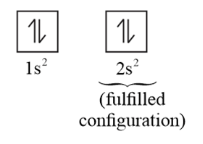Electronic configuration of $\mathrm{B}=1 \mathrm{~s}^{2} 2 \mathrm{~s}^{2} 2 \mathrm{p}^{1}$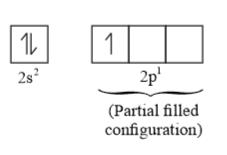During the process of ionization, the electron to be removed from the beryllium atom is a 2 s-electron, whereas the electron to be removed from the boron atom is a 2p-electron. Now, 2 s-electrons are more fulfilled configuration. Therefore, more energy is required to remove a 2s-electron of beryllium than that required to remove a $2 \mathrm{p}$ -electron of boron. Hence, beryllium has a higher enthalpy of ionisation than boron.

(ii) Electronic configuration of Nitrogen $=1 \mathrm{~s}^{2} 2 \mathrm{~s}^{2} 2 \mathrm{p}^{3}$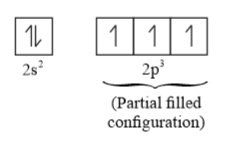Electronic configuration of $\mathrm{Oxygen}=1 \mathrm{~s}^{2} 2 \mathrm{~s}^{2} 2 \mathrm{p}^{4}$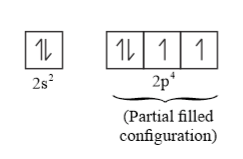The three $2 \mathrm{p}$ -electrons of nitrogen occupy three different atomic orbitals. However, in oxygen, two of the four $2 \mathrm{p}$ -electrons of oxygen occupy the same $2 \mathrm{p}$ -orbital. This results in increased electron-electron repulsion in an oxygen atom. As a result, the energy required to remove the fourth $2 \mathrm{p}$ -electron from oxygen is less as compared to the energy required to remove one of the three $2 \mathrm{p}$ -electrons from nitrogen. Fluorine contains one electron and one proton more than oxygen. As the electron is being added to the same shell, the increase in nuclear attraction (due to the addition of a proton) is more than the increase in electronic repulsion (due to the addition of an electron). Therefore, the valence electrons in fluorine atom experience a more effective nuclear charge than that experienced by the electrons present in oxygen. As a result, more energy is required to remove an electron from fluorine atom than that is required to remove an electron from oxygen atom. Hence, oxygen has a lower $\Delta_{i} \mathrm{H}$ than fluorine.

Question 17. How would you explain the fact that the first ionization enthalpy of sodium is lower than that of magnesium, but its second ionization enthalpy is higher than that of magnesium?

Solution: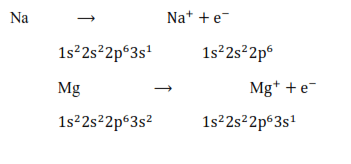The first ionization enthalpy of sodium is more than that of magnesium. This is primarily because of two reasons:

1) The atomic size of sodium is greater than that of magnesium.

2) The effective nuclear charge of magnesium is higher than that of sodium.

For these reasons, the energy required to remove an electron from magnesium is more than the energy required in sodium. Hence, the first ionization enthalpy of sodium is lower than that of magnesium.

$\mathrm{Na}^{+} \quad \rightarrow \mathrm{Na}^{+2}+\mathrm{e}^{-}$

$1 s^{2} 2 s^{2} 2 p^{6} 1 s^{2} 2 s^{2} 2 p^{5}$

$\mathrm{Mg}^{+} \rightarrow \mathrm{Mg}^{+2}+\mathrm{e}^{-}$

$1 \mathrm{~s}^{2} 2 \mathrm{~s}^{2} 2 \mathrm{p}^{6} 3 \mathrm{~s}^{1} \quad 1 \mathrm{~s}^{2} 2 \mathrm{~s}^{2} 2 \mathrm{p}^{6}$

The second ionization enthalpy of sodium is higher than that of magnesium. This is because after losing an electron, sodium attains the stable noble gas configuration, whereas, magnesium, after losing an electron still has one electron in the 3 s-orbital. To attain the nearest stable noble gas configuration, it still has to lose one more electron. Thus, the energy required to remove the second electron in case of sodium is much higher than that required in case of magnesium. Therefore, the second ionization enthalpy of sodium is higher than that of magnesium.

Question 18. What are the various factors due to which the ionization enthalpy of the main group elements tends to decrease down a group?

Solution: The factors responsible for the ionization enthalpy of the main group elements to decrease down a group are listed below:

(i) Increase in the atomic size of elements: As we move down a group, the number of shells increases. As a result, the atomic size also increases gradually on moving down a group. As the distance of the valence electrons from the nucleus increases, the electrons are not held very strongly. Thus, they can be removed easily-Hence, on moving down a group, ionization energy decreases.

(ii) Increase in the shielding effect: The number of inner shells of electrons increases on moving down a group. Therefore, the shielding of the valence electrons from the nucleus by the inner core electrons increases down a group. As a result, the valence electrons are not held very tightly by the nucleus. Hence, the energy required to remove a valence electron decreases down a group.

Question. 19 The first ionization enthalpy values $\mathrm{kJ} \mathrm{mol}^{-1}$ ) of group 13 elements are: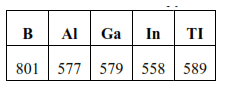How would you explain this deviation from the general trend?

Solution: Generally, when we move down a group, ionization enthalpy decreases due to an increase in the atomic size and shielding.

Ionization energy $\propto \frac{1}{\text { atomic size }}$

Ionization energy $\propto \frac{1}{\text { shielding effect }}$

Thus, on moving down the group ,ionization enthalpy decreases from $\mathrm{B}$ to $\mathrm{A} 1 .$ But, Ge has higher ionization enthalpy than Al. Al follows immediately after s- block elements, whereas Ga follows after d- block elements. The shielding provided by d-electrons is not very effective. These electrons do not shield the valence electrons very effectively. As a result, the valence electrons of $\mathrm{Ga}$ experience a greater effective nuclear charge than those of $\mathrm{Al}$. Further, on moving from Ga to In, the ionization enthalpy decreases due to an increase in the atomic size and shielding. But, on moving from In to $\mathrm{Tl}$, the ionization enthalpy again increases. In the periodic table, Tl follows after $4 \mathrm{f}$ and $5 \mathrm{~d}$ electrons. The shielding provided by the electrons in both these orbitals is not very effective. Therefore, the valence electron is held quite strongly by the nucleus. Hence, the ionization energy of $\mathrm{Tl}$ is on the higher side.

Question. 20 Which of the following pairs of elements would have a more negative electron gain enthalpy?

(i) 0 or $\mathrm{F}$

(ii) $\mathrm{F}$ or $\mathrm{Cl}$

Solution: (i) Electronic configuration of oxygen $: 1 s^{2} 2 s^{2} 2 p^{4}$

Electronic configuration of fluorine : $1 s^{2} 2 s^{2} 2 p^{5}$

0 and $\mathrm{F}$ are present in the same period of the periodic table. An $\mathrm{F}$ atom has one proton and one electron more than 0 and as an electron is being added to the same shell, the atomic size of $\mathrm{F}$ is smaller than that of O. As $\mathrm{F}$ contains one proton more than 0 , its nucleus can attract the incoming electron more strongly in comparison to the nucleus of 0 atom. Also, f needs only one more electron to attain the stable noble gas configuration. Hence, the electron gain enthalpy of $\mathrm{F}$ is more negative than that of $\mathrm{O}$.

(ii) $\mathrm{F}$ and $\mathrm{Cl}$ belong to the same group of the periodic table. The electror gain enthalpy usually becomes less negative on moving down a group. However, in this case, the value of the electron gain enthalpy of $\mathrm{Cl}$ is more negative than that of $\mathrm{F}$. This is because the atomic size of $\mathrm{F}$ is smaller than that of $\mathrm{Cl}$. In $\mathrm{F}$, the electron will be added to quantum level $\mathrm{n}=2$, but in $\mathrm{Cl}$, the electron is added to quantum level $\mathrm{n}=$ 3 and chlorine has a vacant $\mathrm{d}$ orbital. Therefore, there are less electron-electron repulsions in $\mathrm{Cl}$, and an additional electron can be accommodated easily. Hence, the electron gain enthalpy of $\mathrm{Cl}$ is more negative than that of $\mathrm{F}$.

Question 21. Would you expect the second electron gain enthalpy of 0 as positive, more negative or less negative than the first? Justify your answer.

Solution: When an electron is added to 0 atom to form $\mathrm{O}$ - ion energy is released. Thus, the first electron gain enthalpy of 0 is negative.

$0(\mathrm{~g})+\mathrm{e}^{-} \rightarrow 0^{-}(\mathrm{g})$

Whereas, when an electron is added to $0^{-}$ ion to form $0^{2-}$ ion, energy has to be given out to overcome the strong electronic repulsions. Thus, the second electron gain enthalpy of 0 is positive.

$0^{-}(g)+\mathrm{e}^{-1} \rightarrow 0^{2-}(\mathrm{g})$

Question 22. What is the basic difference between the terms electron gain enthalpy and electronegativity?

Solution: Electron gain enthalpy is the measure of the amount of energy of an isolated gaseous atom to accept an electron, whereas electronegativity is the measure of the tendency to attract a shared pair of electrons of an atom during the bond.

Electron gain enthalpy has unit eV/atom or joule/atom and electronegativity is unit less.

Question 23. How would you react to the statement that the electronegativity of $\mathrm{N}$ on the Pauling scale is $3.0$ in all the nitrogen compounds?

Solution: Electronegativity of an element is a variable property. It is different in different compounds. Hence, the statement which says that the electronegativity of $\mathrm{N}$ on the Pauling scale is $3.0$ in all nitrogen compounds is incorrect. For example, the electronegativity of $\mathrm{N}$ is different in $\mathrm{NH}_{3}$ and $\mathrm{NO}_{2}$

Question 24. Describe the theory associated with the radius of an atom as it

(a) gains an electron

(b) loses an electron

Solution: (a) By gaining an electron, an anion is formed.

$A+e^{-} \rightarrow A^{-}$

When an atom gains an electron, its size increases. When an electron is added, the number of electrons goes up by one. This results in an increase in repulsion among the electrons. However, the number of protons remains the same. As a result, the effective nuclear charge of the atom decreases and the radius of the atom increases.

(b) By the loss of an electron, a cation is formed

$B \longrightarrow B^{+}+e^{-}$

When an atom loses an electron, the number of electrons decreases by one while the nuclear charge remains the same. Therefore, the interelectronic repulsions in the atom decreases. As a result, the effective nuclear charge increases. Hence, the radius of the atom decreases.

Question 25. Would you expect the first ionization enthalpies for two isotopes of the same element to be the same or different? Justify your answer

Solution: The ionization enthalpy of an atom depends on the number of electrons and protons (nuclear charge) of that atom. Now, the isotopes of an element have the same number of protons and electrons. Therefore, the first ionization enthalpy for two isotopes of the same element should be the same.

Question 26. What are the major differences between metals and non-metals?

Solution: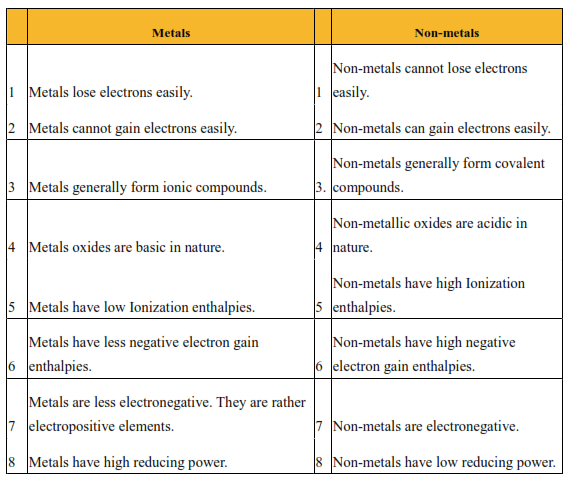Question 27. Use the periodic table to answer the following questions.

(a) Identify an element with five electrons in the outer subshell.

(b) Identify an element that would tend to lose two electrons.

(c) Identify an element that would tend to gain two electrons.

(d) Identify the group having metal, non-metal, liquid as well as gas at the room temperature. Solution: (a) The element has 5 electrons in its outermost subshell means that the electronic configuration should be $\mathrm{ns}^{2} \mathrm{np}^{5}$. This represents the electronic configuration of the halogen group. Thus, the element can be F, Cl, Br, I, or At.

(b) An element that has two valence electrons will tend to lose two electrons easily to attain the nearest stable noble gas configuration. The general electronic configuration of such an element thus will be $\mathrm{ns}^{2}$. This represents the electronic configuration of group 2 elements. Therefore the element can be Be, $\mathrm{Mg}, \mathrm{Ca}, \mathrm{Sr}$, or $\mathrm{Ba}$.

(c) An element tends to gain two electrons if it needs only two electrons to attain the nearest stable noble gas configuration. The general electronic configuration of such an element should be $\mathrm{ns}^{2} \mathrm{np}^{4}$. This represents the electronic configuration of the oxygen family. Therefore the element can be $\mathrm{O}, \mathrm{S}$, Se, Te, or Po.

(d) The group having metal, non-metal, liquid as well as gas at the room temperature is group 17 .

Question 28. The increasing order of reactivity among group 1 elements is $\mathrm{Li}<\mathrm{Na}<\mathrm{K}<$ Rb whereas that among group 17 elements is $C I>B r>I$. Explain.

Solution: The Group 1 elements have only one valence electron, which they tend to lose. Whereas, Group 17 elements need only one electron to attain the nearest noble gas contiguration. On moving down group 1, the ionisation enthalpies decrease which means that the energy required to lose the valence electron decreases. Thus, reactivity increases on moving down a group. Thus, the increasing order of reactivity among group 1 elements is as follows:

$\mathrm{Li}<\mathrm{Na}<\mathrm{K}<\mathrm{Rb}<\mathrm{Cs}$

In group 17, on moving down the group from $\mathrm{Cl}$ to $\mathrm{I}$, the electron gain enthalpy becomes less negative. Thus, the tendency to gain electrons decreases down group 17. So, reactivity decreases down a group. Thus, $\mathrm{F}$ is the most reactive halogen. The decreasing order of reactivity among group 17 elements is as follows:

$\mathrm{F}>\mathrm{Cl}>\mathrm{Br}>\mathrm{I}$

Question 29. Write the general outer electronic configuration of $s-, p-, d-$ and $f-$ block elements.

Solution: The general outer electronic configuration of:

$s-$ block is $\mathrm{ns}^{1-2}$, where $\mathrm{n}=2$ to 7

$p-$ block is $n s^{2} n p^{1-6}$, where $n=2$ to 6

$\mathrm{d}-$ block is $(\mathrm{n}-1) \mathrm{d}^{1-10} \mathrm{~ns}^{0-2}$, where $\mathrm{n}=4$ to 7

$\mathrm{f}-$ block is $(\mathrm{n}-2) \mathrm{f}^{1-14}(\mathrm{n}-1) \mathrm{d}^{0-10} \mathrm{~ns}^{2}$, where $\mathrm{n}=6$ to 7

Question 30. Assign the position of the element having the outer electronic configuration

(i) $\mathrm{ns}^{2} \mathrm{np}^{4}$ for $\mathrm{n}=3$

(ii) $\quad(\mathrm{n}-1) \mathrm{d}^{2} \mathrm{~ns}^{2}$ for $\mathrm{n}=4$, and

(iii) $\quad(\mathrm{n}-2) \mathrm{f}^{7}(\mathrm{n}-1) \mathrm{d}^{1} \mathrm{~ns}^{2}$ for $\mathrm{n}$

$=6$, in the periodic table.

Solution: (i) $\mathrm{n}=3$ means that the element belongs to the 3rd period. It is a p-block element because the last electron occupies the $\mathrm{p}$ - orbital. There are four electrons in the p-orbital.

So, the corresponding group of the element $=$ Number of s-block groups $+$ the number of $d$ -block groups $+$ the number of $\mathrm{p}$ -electrons $=2+10+4=16$

Hence, the element belongs to the 3rd period and the 16 th group of the periodic table. Therefore, the element is Sulphur.

(ii) The element belongs to the 4 th period since $\mathrm{n}=4 .$ It is a d-block element as $\mathrm{d}-$ orbitals are not completely filled. There are 2 electrons in the d- orbital.

So, the corresponding group of the element $=$ Number of $s$ -block groups $+$ the number of d-block groups $=2+2=4$

Hence, the element belongs to the 4 th period and the 4 th group.

Therefore, the element is Titanium.

(iii) The element belongs to the 6 th period since $\mathrm{n}=6$. It is an $\mathrm{f}$ - block element as the last electron occupies the $\mathrm{f}$ - orbital. It belongs to group 3 of the periodic table because all $\mathrm{f}$ -block elements belong to group $3 .$ Its electronic configuration is $[\mathrm{Xe}] 4 \mathrm{f}^{7} 5 \mathrm{~d}^{1} 6 \mathrm{~s}^{2}$. Thus, its atomic number is $54+7+1+2=64$. Therefore, the element is Gadolinium.

Question 31. The first $\Delta_{\mathrm{i}} \mathrm{H}_{1}$ and the second $\Delta_{\mathrm{i}} \mathrm{H}_{2}$ ionisation enthalpies (in $\mathrm{kJ} \mathrm{mol}^{-1}$ ) and the $\Delta_{\mathrm{eq}} \mathrm{H}$ electron gain enthalpy in $\left(\mathrm{kJ} \mathrm{mol}^{-1}\right)$ of a few elements are given below: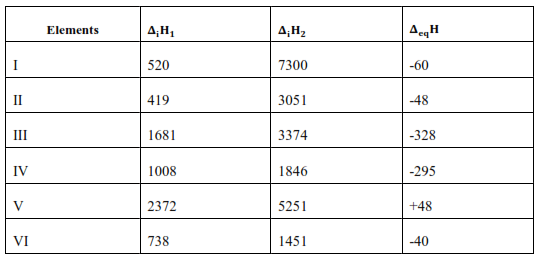Which of the above elements is likely to be:

(a) the least reactive element.

(b) the most reactive metal.

(c) the most reactive non-metal.

(d) the least reactive non-metal.

(e) the metal which can form a stable binary halide of the formula $\mathrm{MX}_{2}(\mathrm{X}=$ halogen $)$

(f) the metal which can form a predominantly stable covalent halide of the formula MX $(\mathrm{X}=$ halogen $) ?$

Solution: (a) Element $\mathrm{V}$ is likely to be the least reactive element since it has the highest first ionisation enthalpy $\Delta_{\mathrm{i}} \mathrm{H}_{1}$ and also a positive electron gain enthalpy $\Delta_{\mathrm{eg}} \mathrm{H}$.

(b) Element II is likely to be the most reactive metal since it has the lowest first ionisation enthalpy $\Delta_{i} \mathrm{H}_{1}$ and a low negative electron gain enthalpy $\Delta_{\text {eg }} \mathrm{H}$.

(c) Element II is likely to be the most reactive nonmetal as has a high first ionisation enthalpy $\Delta \mathrm{H}_{1}$ and the highest negative electron gain enthalpy $\Delta_{\text {oo }} \mathrm{H}$.

(d) Element $\mathrm{V}$ is likely to be the least reactive non-metal since it has a very high first ionisation enthalpy $\Delta_{\mathrm{i}} \mathrm{H}_{2}$ and a positive electron gain enthalpy $\Delta_{\text {eg }} H$.

(e) Element VI has a low negative electron gain enthalpy $\Delta_{\mathrm{eg}} \mathrm{H} .$ Thus, it is a metal. Further, it has the lowest second ionisation enthalpy $\Delta_{\mathrm{i}} \mathrm{H}_{2}$. Hence, it can form a stable binary halide of the formula $\mathrm{MX}_{2}$ (Where $\mathrm{X}$ is a halogen).

(f) Element I has low first ionisation energy and high second ionisation energy. Hence, it can form a predominantly stable covalent halide of the formula $\mathrm{MX}(\mathrm{X}=$ halogen $)$.

Question 32. Predict the formula of the stable binary compounds that would be formed by the combination of the following pairs of elements.

(a) Lithium and oxygen

(b) Magnesium and nitrogen

(c) Aluminium and iodine

(d) Silicon and oxygen

(e) Phosphorus and fluorine

(f) Element 71 and fluorine

Solution. (a) $\quad$ Lithium dioxide $-\mathrm{LiO}_{2}$

(b) Magnesium nitrite $-\mathrm{Mg}_{3} \mathrm{~N}_{2}$

(c) Aluminium triiodide $-\mathrm{AlI}_{3}$

(d) Silicon dioxide $-\mathrm{SiO}_{2}$

(e) Phosphorous trifluoride $-\mathrm{PF}_{3}$ or Phosphorus pentafluoride $-\mathrm{PF}_{5}$

(f) The element with atomic number 71 is Lutetium (Lu). It has valency 3 Therefore, the formula of the compound is $\mathrm{LuF}_{3}$.

Question 33. In the modern periodic table, the period indicates the value of:

(a) atomic number

(b) atomic mass

(c) principal quantum number

(d) azimuthal quantum number

Solution. Option (c) is correct.

The value of the principal quantum number (n) for the outermost shell or the valence shell indicates a period in the Modern periodic table.

Question 34. Which of the following statements related to the modern periodic table is incorrect?

(a) The p-block has 6 columns because a maximum of 6 electrons can occupy all the orbitals in a p-shell.

(b) The d-block has 8 columns because a maximum of 8 electrons can all the orbitals in a d-subshell.

(c) Each block contains a number of columns equal to the number of electrons that can occupy that subshell.

(d) The block indicates the value of the azimuthal quantum number (l) for the last subshell that received electrons in building up the electronic configuration

Solution. Statement (b) is incorrect. The $d$ -block has 10 columns because a maximum of 10 electrons can occupy all the orbitals in ad subshell.

Question 35. Anything that influences the valence electrons will affect the chemistry of the element. Which one of the following factors does not affect the valence shell?

(a) Valence principal quantum number (n)

(b) Nuclear charge $(\mathrm{Z})$

(c) Nuclear mass

(d) Number of core electrons.

Solution: The nuclear mass does not affect the valence shell. Hence option (c) is correct.

Question 36. The size of isoelectronic species $\Gamma, \mathrm{Ne}$ and $\mathrm{Na}^{+}$ is affected by

(a) nuclear charge ( $\mathrm{Z}$ )

(b) valence principal quantum number (n)

(c) electron-electron interaction in the outer orbitals

(d) none of the factors because their size is the same.

Solution: The size of an isoelectronic species increases with a decrease in the nuclear

charge (Z). For example, the order of the increasing nuclear charge of $\mathrm{F}^{-}$, Ne, and $\mathrm{Na}^{+}$ is as follows:

$\mathrm{F}^{-}<\mathrm{Ne}<\mathrm{Na}^{+}$

$\begin{array}{llll}Z & 9 & 10 & 11\end{array}$

Therefore, the order of the increasing size of is as follows: $\mathrm{Na}^{+}<\mathrm{Ne}<\mathrm{F}^{-}$

Question 37. Which one of the following statements is incorrect in relation to ionization enthalpy?

(a) Ionisation enthalpy increases for each successive electron.

(b) The greatest increase in ionisation enthalpy is experienced on the removal of an electron from the core noble gas configuration.

(c) End of valence electrons is marked by a big jump in ionisation enthalpy.

(d) Removal of electron from orbitals bearing lower $\mathrm{n}$ value is easier than from orbital having higher $\mathrm{n}$ value.

Solution. Electrons in orbitals having a lower $\mathrm{n}$ value are more attracted to the nucleus than electrons in orbitals having a higher $\mathrm{n}$ value. Hence, the removal of electrons from orbitals bearing a higher $\mathrm{n}$ value is easier than the removal of electrons from orbitals having a lower n value. Therefore, the correct option is $(\mathrm{d})$

Question 38. Considering the elements $\mathrm{B}, \mathrm{Al}, \mathrm{Mg}$, and $\mathrm{K}$, the correct order of their metallic character is:

(a) $\quad \mathrm{B}>\mathrm{Al}>\mathrm{Mg}>\mathrm{K}$

(b) $\quad \mathrm{Al}>\mathrm{Mg}>\mathrm{B}>\mathrm{K}$

(c) $\quad \mathrm{Mg}>\mathrm{A} 1>\mathrm{K}>\mathrm{B}$

(d) $\mathrm{K}>\mathrm{Mg}>\mathrm{Al}>\mathrm{B}$

Solution. The metallic character of elements decreases as we from left to right across a period. So, the metallic character of $\mathrm{Mg}$ is more than that of Al. Also, the metallic character of elements increases down a group. So, the metallic character of $\mathrm{Al}$ is more than that of $\mathrm{B}$. From the above statements, we get $K>M g .$ Hence, the correct order of metallic character is $\mathrm{K}>\mathrm{Mg}>\mathrm{Al}>\mathrm{B}$.

Question 39. Considering the elements $\mathrm{B}, \mathrm{C}, \mathrm{N}, \mathrm{F}$, and $\mathrm{Si}$, the correct order of their non-metallic character is:

(a) $\quad \mathrm{B}>\mathrm{C}>\mathrm{Si}>\mathrm{N}>\mathrm{F}$

(b) $\quad \mathrm{Si}>\mathrm{C}>\mathrm{B}>\mathrm{N}>\mathrm{F}$

(c) $\quad \mathrm{F}>\mathrm{N}>\mathrm{C}>\mathrm{B}>\mathrm{Si}$

(d) $\quad \mathrm{F}>\mathrm{N}>\mathrm{C}>\mathrm{Si}>\mathrm{B}$

Solution. The non-metallic character of elements increases as we move from left to right across a period. Thus, the decreasing order of non-metallic character is $\mathrm{F}\rangle$ $\mathrm{N}>\mathrm{C}>\mathrm{B}$. Also, the non-metallic character of elements decreases on moving down a group. Thus, the decreasing order of non-metallic characters of $\mathrm{C}$ and Si are $C>$ Si. However, $S i$ is less nonmetallic than B, i.e., B > Si. Hence, the correct order of their non-metallic characters is $\mathrm{F}>\mathrm{N}>\mathrm{C}>\mathrm{B}>\mathrm{Si}$.

Question 40. Considering the elements $\mathrm{F}, \mathrm{Cl}, 0$ and $\mathrm{N}$, the correct order of their chemical reactivity in terms of oxidizing property is:

(a) $\quad \mathrm{F}>\mathrm{Cl}>0>\mathrm{N}$

(b) $\quad \mathrm{F}>0>\mathrm{Cl}>\mathrm{N}$

(c) $\quad \mathrm{Cl}>\mathrm{F}>0>\mathrm{N}$

(d) $\quad 0>\mathrm{F}>\mathrm{N}>\mathrm{Cl}$

Solution: The oxidizing character of elements increases from left to right across a period. Thus, we get the decreasing order of oxidizing property as $\mathrm{F}>0>\mathrm{N}$. Again, the oxidizing character of elements decreases down a group. Thus, we get $\mathrm{F}\rangle$ Cl. However, the oxidizing character of 0 is more than that of $C l$ i.e., $0>$ Cl. Hence, the correct order of chemical reactivity of $\mathrm{F}, \mathrm{Cl}, 0$, and $\mathrm{N}$ in terms of their oxidizing property is $\mathrm{F}>0>\mathrm{Cl}>\mathrm{N}$.